Courses

# CPU - Redox Reaction - Retro JEE Main

## 9 Questions MCQ Test | CPU - Redox Reaction - Retro JEE Main

Description
This mock test of CPU - Redox Reaction - Retro JEE Main for Class 11 helps you for every Class 11 entrance exam. This contains 9 Multiple Choice Questions for Class 11 CPU - Redox Reaction - Retro JEE Main (mcq) to study with solutions a complete question bank. The solved questions answers in this CPU - Redox Reaction - Retro JEE Main quiz give you a good mix of easy questions and tough questions. Class 11 students definitely take this CPU - Redox Reaction - Retro JEE Main exercise for a better result in the exam. You can find other CPU - Redox Reaction - Retro JEE Main extra questions, long questions & short questions for Class 11 on EduRev as well by searching above.
QUESTION: 1

### Retro JEE Main  (Compilation of Last 13 Years  Questions) IIT JEE (Main & Advanced) past year questions with solutions & answer key Retro IIT JEE (Main and Advanced) have questions from previous/past (10 to 13 years) papers of IIT JEE (Main and Advanced) with solutions, The following set of questions contain previous/past (10 to  13 years) questions of IIT JEE (Main and Advanced) of Chapter "Redox Reaction" with solutions  Q. In which of the following reactions H2O2 acts as a reducing agent?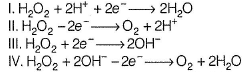Solution:

A species which is oxidised (de-electronation) is a reducing agent.
(I) Electronation — H2O2 is reduced
(II) De - electronation — H2O2 is oxidised (III) Electronation — H2O22 is reduced
(IV) De - electronation — H2O2 is oxidised
Thus, (II) and (IV) representreducing behaviour of H2O2.

QUESTION: 2

### Consider the following reaction,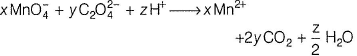The values of x, y and z in the reaction are, respectively (AIEEE 2013)

Solution:

The half equations of the reaction are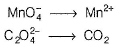The balanced half equations are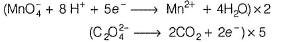Equating number of electrons, we get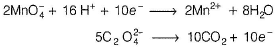On adding both the equations, we get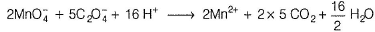Thus, x, y and z are 2, 5 and 16, respectively.

QUESTION: 3

### Which of the following chemical reactions depicts the oxidising behaviour of H2SO4? (AIEEE 2006)

Solution: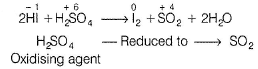QUESTION: 4

The oxidation state of Cr in [Cr(NH3)4CI2]+ is

[AIEEE 2005]

Solution: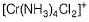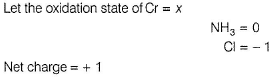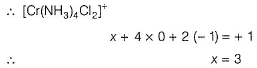QUESTION: 5

The oxidation state of chromium in the final product formed by the reaction between Kl and acidified potassium dichromate solution is

(AIEEE 2005)

Solution: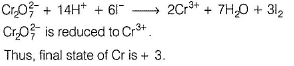QUESTION: 6

Oxidation number of Cl in CaOCI2 (bleaching powder) is

(AIEEE 2002)

Solution: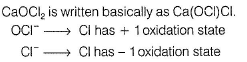QUESTION: 7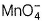is a good oxidising agent in different medium changing to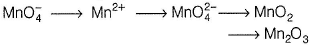Changes in oxidation number respectively, are

(AIEEE 2002)

Solution: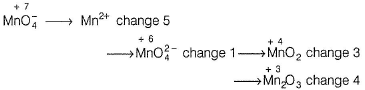QUESTION: 8

Which of the following is a redox reaction ?

(AIEEE 2002)

Solution: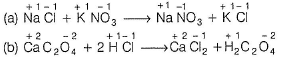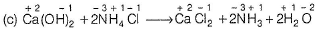In all these cases during reaction, there is no change in oxidation state of ion or molecule or constituent atom. These are simply ionic reactions.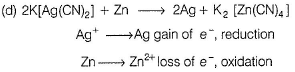QUESTION: 9

Which of the following reaction is possible at anode ?

(AIEEE 2002)

Solution:

Oxidation takes place at anode. Hence, (a), (b) is not feasible as these are reduction reactions.'But Cr3+ is oxidised to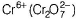under given condition.